## 前言

Hyperconnect 开发了一款名为 Azar 的移动应用，在世界上有很多用户.Figure 1. 移动设备上网络的快速运行示例. 在 Pixel 1 上可以达到单次推断 6ms；在 Galaxy J7 上单次推断 28 ms. 完整 Video.

•  - 理解 TensorFlow 和 TensorFlow Lite 的实现细节
•  - 设计神经网络，充分利用 TensorFlow Lite 的 optimized kernels (参考章节 1, 2, 3).
•  - 修改 TOCO: TensorFlow Lite Optimizing Converter 以正确转换未支持的网络层(参考章节 4).
•  - 采用 SIMD 优化代码，加速 quantized-layers (参考章节 5, 6).

## 1. Tensorflow Lite 中的深度可分离层(depthwise separable layer) 为什么速度快？

low-level programs 的实现需要一些不同的思想和方法.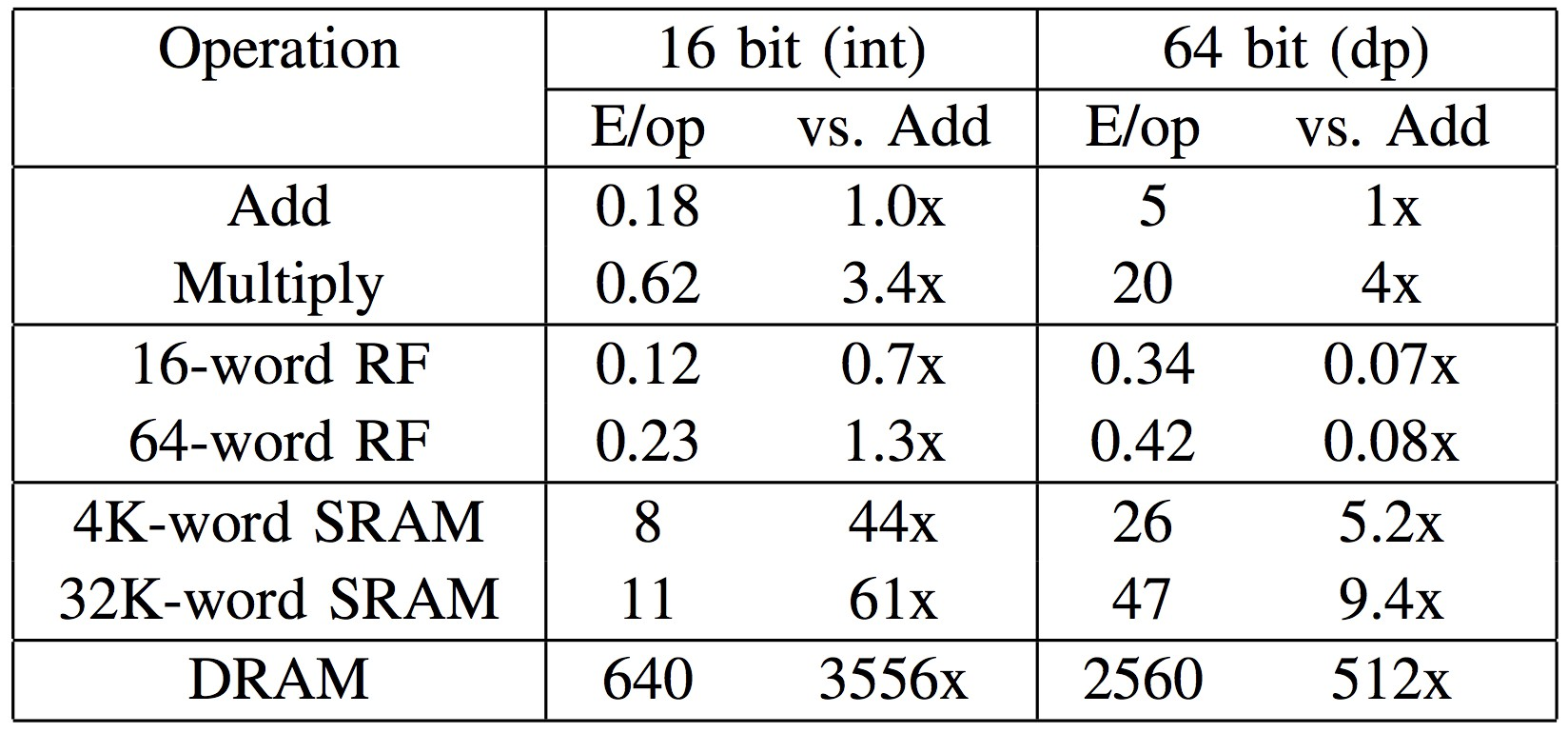Figure 2. 内存访问比加法或乘法需要更多的资源(640 pJ). 访问 cache memory (8 pJ) 比使用 main memory 资源占用少很多.[图片来自 Ardavan Pedram]

### 1.1 Loop Unrolling

for (int i = 0; i < 32; i++) {
x[i] = 1;
if (i%4 == 3) x[i] = 3;
}


for (int i = 0; i < 8; i++) {
x[4*i  ] = 1;
x[4*i+1] = 1;
x[4*i+2] = 1;
x[4*i+3] = 3;
}


### 1.2 Separate implementation for each case

template <int kFixedOutputY, int kFixedOutputX, int kFixedStrideWidth, int kFixedStrideHeight>
struct ConvKernel3x3FilterDepth8 {};


TensorFlow Lite 进一步指定了不同 strides，输出width 和 height 的 16 种情况的实现，

template <> struct ConvKernel3x3FilterDepth8<8, 8, 1, 1> { ... }
template <> struct ConvKernel3x3FilterDepth8<4, 4, 1, 1> { ... }
template <> struct ConvKernel3x3FilterDepth8<4, 2, 1, 1> { ... }
template <> struct ConvKernel3x3FilterDepth8<4, 1, 1, 1> { ... }
template <> struct ConvKernel3x3FilterDepth8<2, 2, 1, 1> { ... }
template <> struct ConvKernel3x3FilterDepth8<2, 4, 1, 1> { ... }
template <> struct ConvKernel3x3FilterDepth8<1, 4, 1, 1> { ... }
template <> struct ConvKernel3x3FilterDepth8<2, 1, 1, 1> { ... }
template <> struct ConvKernel3x3FilterDepth8<4, 2, 2, 2> { ... }
template <> struct ConvKernel3x3FilterDepth8<4, 4, 2, 2> { ... }
template <> struct ConvKernel3x3FilterDepth8<4, 1, 2, 2> { ... }
template <> struct ConvKernel3x3FilterDepth8<2, 2, 2, 2> { ... }
template <> struct ConvKernel3x3FilterDepth8<2, 4, 2, 2> { ... }
template <> struct ConvKernel3x3FilterDepth8<2, 1, 2, 2> { ... }
template <> struct ConvKernel3x3FilterDepth8<1, 2, 2, 2> { ... }
template <> struct ConvKernel3x3FilterDepth8<1, 4, 2, 2> { ... }


### 1.3 智能内存访问模式 Smart Memory Access Pattern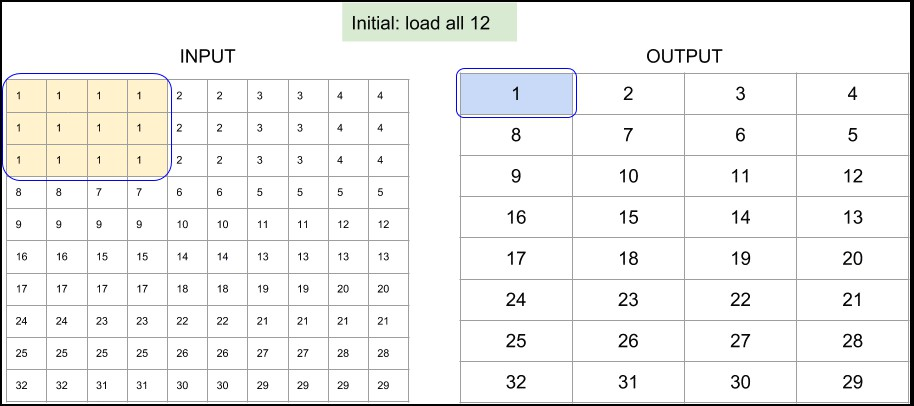Figure 3. TensorFlow Lite 的 depthwise 3x3 conv 实现中，8x8 输出和单位步长的内存访问模式.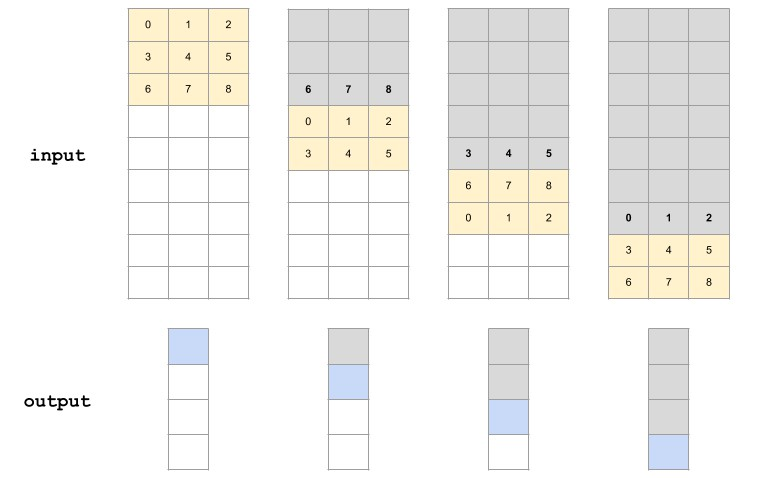Figure 4. TensorFlow Lite 的 depthwise 3x3 conv 实现中，4x1 输出和步长为2的内存访问模式.(加粗变量被重用)

## 2. atrous depthwise convolution 的理解

Atrous Conv 对于图像分割很有帮助. 该项目也会应用到.

rate: 1-D of size 2.
The dilation rate in which we sample input values across the height and width dimensions in atrous convolution.
If it is greater than 1, then all values of strides must be 1.


>>> import tensorflow as tf
>>> tf.enable_eager_execution()
>>> input_tensor = tf.constant(list(range(64)), shape=[1, 8, 8, 1], dtype=tf.float32)
>>> filter_tensor = tf.constant(list(range(1, 10)), shape=[3, 3, 1, 1], dtype=tf.float32)
>>> print(tf.nn.depthwise_conv2d(input_tensor, filter_tensor,
strides=[1, 2, 2, 1], padding="VALID", rate=[2, 2]))

tf.Tensor(
[[[[ 302.] [ 330.] [ 548.] [ 587.]]
[[ 526.] [ 554.] [ 860.] [ 899.]]
[[1284.] [1317.] [1920.] [1965.]]
[[1548.] [1581.] [2280.] [2325.]]]], shape=(1, 4, 4, 1), dtype=float32)

>>> 0 * 5 + 2 * 6 + 16 * 8 + 9 * 18   # The value in (0, 0) is correct
302
>>> 0 * 4 + 2 * 5 + 4 * 6 + 16 * 7 + 18 * 8 + 20 * 9   # But, the value in (0, 1) is wrong!
470


## 3. 高效语义分割网络的设计原则

Tensorflow Lite 首先加载 8 的倍数，然后分别是 4, 2 和 1 的倍数. 因此，最佳的是，保持卷积层的每个输入的尺寸为 8 的倍数.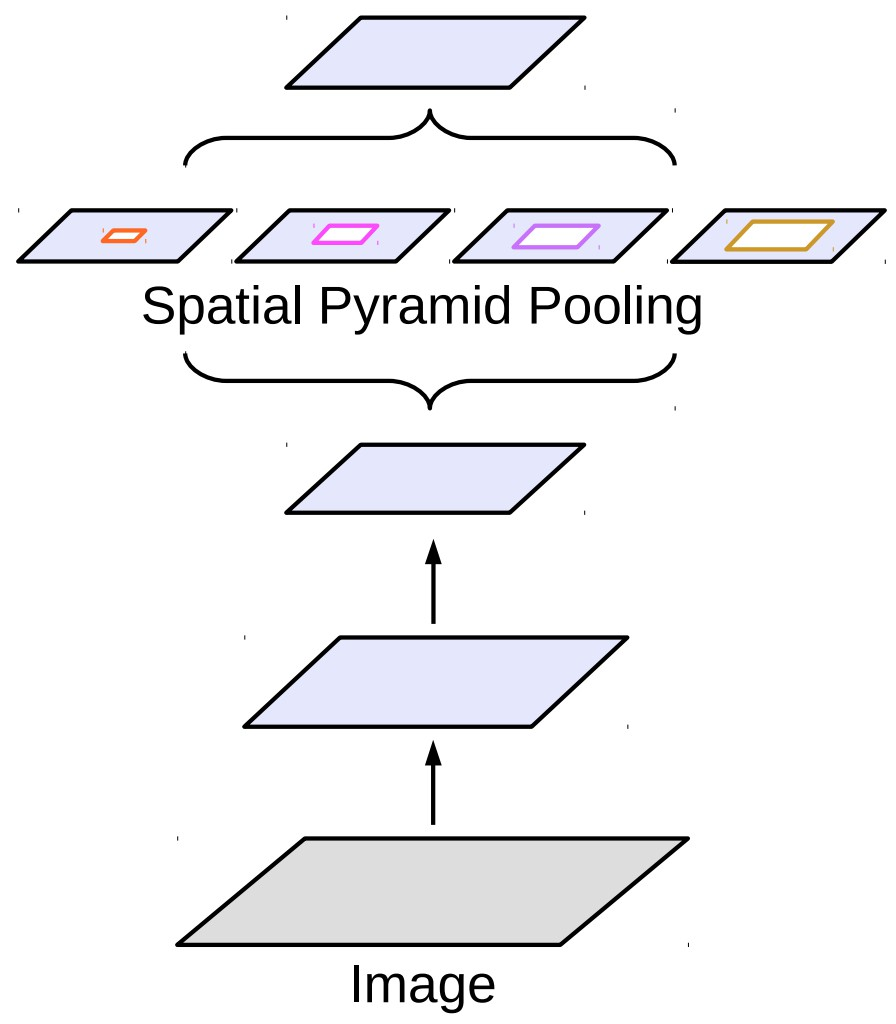Figure 5. Atrous spatial pyramid pooling, ASPP

ASPP 整合了 pyramid pooling 和 atrous conv 操作. DeepLab 在最后一层采用了 ASPP.

## 4. 将 batchnorm 折叠进 atrous depthwise convolution

TensorFlow 的 slim.separable_convolution2d 中，atrous depthwise convolution 的实现是通过将 SpaceToBatchNDSpaceToSpaceND 操作添加到 normal depthwise convolution 中.

SpaceToBatchND → DepthwiseConv2dNative → BatchToSpaceND → BatchNorm


Timing log from the experiment on Galaxy S8
...
[DepthwiseConv] elapsed time: 34us
...


SpaceToBatchND → DepthwiseConv2dNative → BatchNorm → BatchToSpaceND


## 5. SIMD-optimized implementation for quantized resize bilinear layer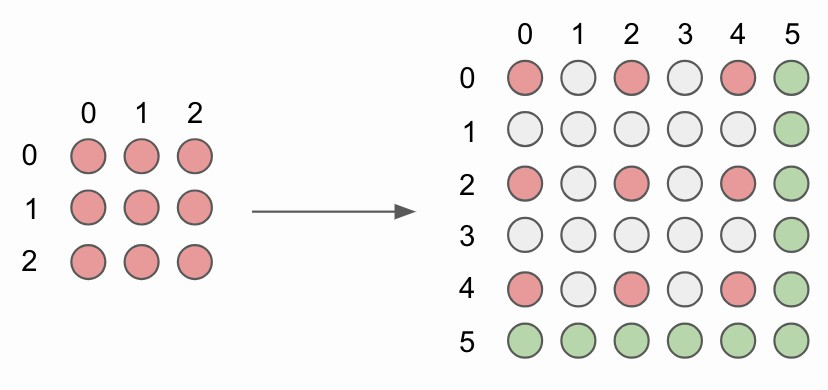Figure 6. 没有角对齐(corner alignment) 的 2x2 双线性上采样.

for (int b = 0; b < batches; b++) {
for (int y0 = 0, y = 0; y <= output_height - 2; y += 2, y0++) {
for (int x0 = 0, x = 0; x <= output_width - 2; x += 2, x0++) {
int32 x1 = std::min(x0 + 1, input_width - 1);
int32 y1 = std::min(y0 + 1, input_height - 1);
ResizeBilinearKernel2x2(x0, x1, y0, y1, x, y, depth, b, input_data, input_dims, output_data, output_dims);
}
}
}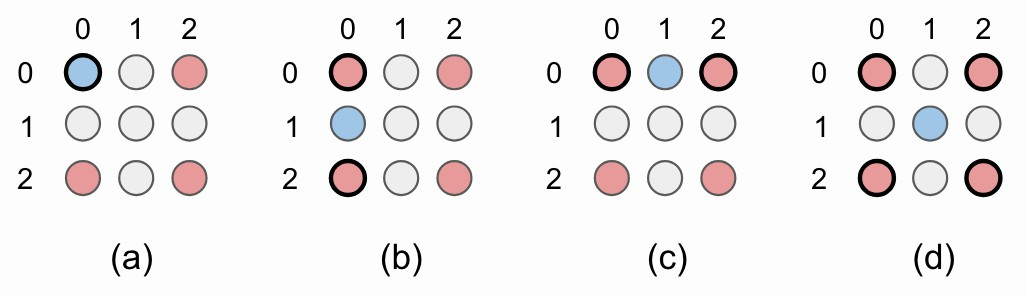Figure 7. 图像的左上角的 2x2 上采样例示. (a) 原来的像素值被重用；(b)-(d) 用于插值新像素值. 红色圈表示原来的像素值. 蓝色圈是新插值的像素值，由黑色圆圈表示的像素值计算得到.

NEON (Advanced SIMD) intrinsics 可以单指令一次处理多个数据. 由于项目处理的 uint8 输入值，可以保存数据为 uint8x16_t, uint8x8_tuint8_t 格式，分别表示 16, 8 和 1 uint8 值. 这种表示可以一次跨多个通道插值像素值.

// Handle 16 input channels at once
int step = 16;
for (int ic16 = ic; ic16 <= depth - step; ic16 += step) {
...
ic += step;
}

// Handle 8 input channels at a once
step = 8;
for (int ic8 = ic; ic8 <= depth - step; ic8 += step) {
...
ic += step;
}

// Handle one input channel at once
for (int ic1 = ic; ic1 < depth; ic1++) {
...
}


quantized bilinear upsampling 的 SIMD 实现是很直接的. 左上角的像素值被重用(Fig 7a)，左下(Fig 7b) 和右上(Fig 7c) 的像素值是两个原来临近像素值的均值. 最后，右下像素值(Fig 7d) 是 4 个原来对角线临近像素值的均值.

// Bottom right
output_ptr += output_x_offset;
vst1q_u8(output_ptr, bottom_right_interpolation);


## 6. Softmax 层和 demo 代码中易出现的错误

TensorFlow Lite 推断时的第一印象是比较慢. 在 Galaxy J7 上耗费 85 ms.

<T> T copyTo(T dst) {
...
// This is just example, of course, hardcoding output shape here is a bad practice
// In our actual app, we build our own JNI interface with just using c++ code
// int[] dstShape = NativeInterpreterWrapper.shapeOf(dst);
int[] dstShape = {1, width*height*channel};
...
}


TensorFlow Lite 中 Softmax 层的实现是对分类任务优化的，然后在 depth 上进行循环，而不是 outer_size. 这就会导致在分割网络中使用 softmax 层推断时间较慢.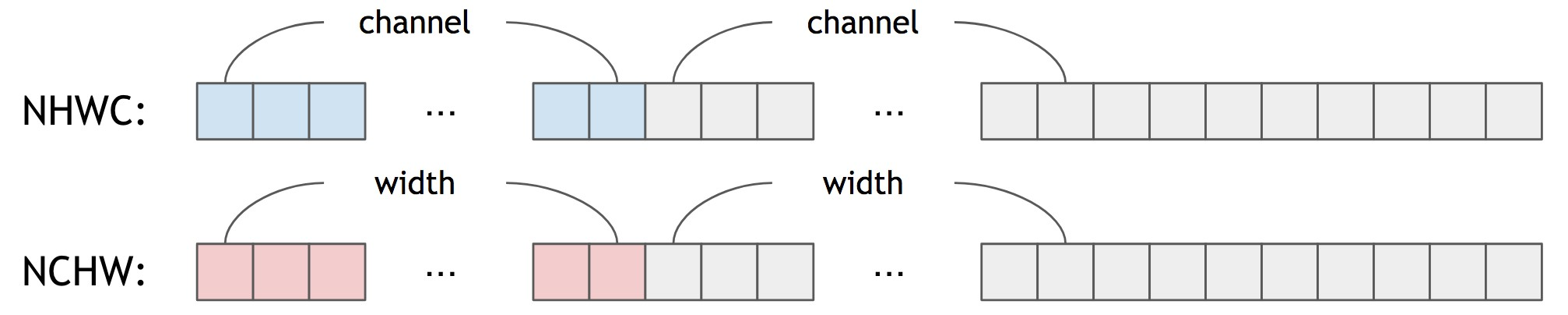Figure 8. NHWC vs NCHW

for (int fg = 0; fg < 256; fg++) {
for (int bg = 0; bg < 256; bg++) {
// Dequantize
float fg_real = input->params.scale * (fg - input->params.zero_point);
float bg_real = input->params.scale * (bg - input->params.zero_point);

// Pre-calculating Softmax Values
...

// Quantize
precalculated_softmax[x][y] = static_cast<uint8_t>(clamped);
}
}


## 参考文献

Last modification：October 9th, 2018 at 09:31 am